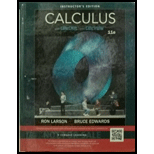Chapter 3.5, Problem 32E### Calculus (MindTap Course List)

11th Edition
Ron Larson + 1 other
ISBN: 9781337275347

#### Solutions

Chapter
Section### Calculus (MindTap Course List)

11th Edition
Ron Larson + 1 other
ISBN: 9781337275347
Textbook Problem

# Finding a Limit In Exercises 17-36, find the limit, if it exists. lim x → ∞ 2 x ( x 6 − 1 ) 1 / 3

To determine

To calculate: The limit limx2x(x61)13 if it exists.

Explanation

Given: limx2x(x61)13.

Formula used: limxp(x)q(x)=limxp(x)limxq(x).

Calculation:

Consider,

limx2x(x61)13=limx2x(x6

### Still sussing out bartleby?

Check out a sample textbook solution.

See a sample solution

#### The Solution to Your Study Problems

Bartleby provides explanations to thousands of textbook problems written by our experts, many with advanced degrees!

Get Started

## Additional Math Solutions

#### Rationalize Numerator Rationalize the numerator. 96. x+1x

Precalculus: Mathematics for Calculus (Standalone Book)

#### Convert the expressions in Exercises 6584 to power form. xy23

Finite Mathematics and Applied Calculus (MindTap Course List)

#### Think About It Explain why In ex=x.

Calculus: Early Transcendental Functions

#### In problems 23-58, perform the indicated operations and simplify. 43.

Mathematical Applications for the Management, Life, and Social Sciences